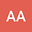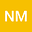Stochastic maximum principle for discrete time mean-field optimal control problems
••Universitat Duisburg-Essen Fakultat fur Mathematik Campus Essen

Corresponding Author:[email protected]

Author Profile## Abstract

In this paper, we study the optimal control of a discrete-time stochastic differential equation (SDE) of mean-field type, where the coefficients can depend on both a function of the law and the state of the process. We establish a new version of the maximum principle for discrete-time mean-field type stochastic optimal control problems. Moreover, the cost functional is also of the mean-field type. This maximum principle differs from the classical principle one since we introduce new discrete-time mean-field backward (matrix) stochastic equations. Based on the discrete-time mean-field backward stochastic equations where the adjoint equations turn out to be discrete backward SDEs with mean field, we obtain necessary first-order and sufficient optimality conditions for the stochastic discrete mean-field optimal control problem. To verify, we apply the result to production and consumption choice optimization problem.
14 Jan 2023Submitted to Optimal Control, Applications and Methods
14 Jan 2023Submission Checks Completed
14 Jan 2023Assigned to Editor
14 Jan 2023Review(s) Completed, Editorial Evaluation Pending
17 Jan 2023Reviewer(s) Assigned
26 Feb 2023Editorial Decision: Revise Minor